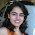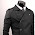## Monday, April 28, 2008

### What would the best base be?

(A partial continuation of the last post).

We use Base 10 because we have 10 fingers on our hands. But if we could pick a base based on what is better mathematically or computationally or some objective criteria, what would it be?
1. When I was young I thought that if we had always used base 8 then computer science would be easier and computers would be faster. While partially true, not MUCH easier or MUCH faster.
2. In 1934 there was an article with title An Excursion in Numbers, by F. Emerson Andrews, in The Atlantic Monthly urged abanding Base 10 for Base 12. (Yes- the The Atlantic Monthly not The American Mathematically Monthly. I'm surprised too.) There are some advantages- 12 is divisible by 2,3,4,6 and since 12 is used for eggs there may have been some reason for it. The Duodecimal Society advocates changing to base 12. They have (or perhaps had - I could not find it on the web) a newsletter The Duodecimal Bulletin, which is translated into one other languauge and has the title Ekskurso en Nombroj. I'll let you figure out what language that is. (ACK- this info comes from Mathematical Cranks by Underwood Dudley.)
3. Picture that you want to represent every number between 1 and n. Lets say its in base 10. In an adding machine (whats that?) you would have log10 n columns and each one of them has 10 keys. So the total number of keys you need is 10log10n. More generally, if its base b then you need blogb keys. What value of b minimizes this? The answer is e. Since we can't use e for normal counting, this does indicate that 2 or 3 would be best. Since 2 is also good for computer science, my vote goes to using base 2.
4. To end where we began this- I wonder how Obama, Hillary, and McCain would vote?

1.Strictly speaking, when minimising the number of keys of the adding machine, the best choice would be 3, with 2 and 4 as runners up.

2.For the theorist, I would think base 1 would clearly be the superior choice. Many intractable problems become quite easy once you assume adopt a unary representation for numbers.

1.how would you represent numbers if the only digit you had was 0?

2.You have the number of digits be the number.

3.Only good in some theoretical situations, but is largely impractical both for mathematics and computers. Normal maths is obvious (write 1 million, anyone?) and computers would simply not work (on, off).

3.Clocks are base 12 and pounds used to be 12 schillings. The large number of factors is a clear advantage. But somehow the latter was dropped. It might happen that hours might have 100 minutes in a few decades.

1.A pound was actually twenty shillings. A shilling was twelve pence.

2.1 hour to be comprised of 100 minutes is already a tried and given up amendment during the French Revolution.

4.Ne surprizas min ke la samaj personoj kiu kredas en la lingvo internacia ankaux kredas en la ebleco de remeto de dekuma sistemo. A, idealistoj...

5.French seems to be a base 20 (vigesimal) language. 80 is 4-20s.
81 is 4-20s+1. 90 is 4-20s+11. Apparently the French still didn't have shoes and were still using their toes when they invented their counting system.

http://en.wikipedia.org/wiki/Vigesimal

6.Minimizing keys is not necessarily the goal. The goal should be to optimize input. To only use two keys when you have ten fingers seems silly, using only ten fingers when there are 20 digits available is surely self-defeeting.

7.Kiel la uzo de la dekdu-sistemo estas idealista? Du (la binara), kompreneble; kvar aux ok, compreneble. Sed ne dekdu !

8.In case it's not clear, the language of translation is Esperanto.

www.cs.auckland.ac.nz/~mcw/blog/

9.I think there are some irrational bases that have nice features, base \phi in particular. Know anyone born with 1.61803398... fingers?

Here is a blog item by Burton McKenzie on the same subject that delves into the irrational-base issue, and Wikipedia has a large page on base \phi. I was interested in whether arithmetic might be sped up enough in such bases as to be worth a one-time overhead of translating binary numbers to it and back.

10.whatever number system we use has to be natural for humans. 2,3 are too small (takes more than twice the digits to represent a base 10 number in binary). 12 is interesting but when we cant even switch to dvorak keyboards, such a switch is not likely to happen, ever.

11.As Ron Paul has as much as much of a chance as Hillary you might as well mention him too. Looks like Nevada had their head on straight this weekend: http://www.lasvegassun.com/news/2008/apr/27/ron-paul-campaign-dominates-convention/

12.13.Base 12 has been known to frighten small children. Just ask the "Schoolhouse Rock" team.

14.What about using *balanced* base 3? That has some nice properties.

Regarding base 12, I was told by an Indian physicist that she was taught to count to twelve on one hand using the three segments on each of her four fingers.

15.12 is the way to go ... the key is the highly composite numbers, more factors means division tends to result in shorter fractional part (3.4 in base-12, vs 3.3333... in base-10).

Also, using the 3 segments x 4 fingers (thumb as the pointer), you can count to 144 on your two hands.

I've been meaning to do a longer post about this on my own blog, but figure I might as well post the spoiler here. Again: highly composite numbers ... maybe we should go for base-24 (or higher!) if it's not too hard to keep track of the digits.

1.16.Everyone espousing base 12:

We have digits 0123456789
what are the other two?

Don't tell me A and B. I think that the A-F in hex cause confusions because our brains try to parse them as words: What is FACE+FADE+EFFACE? While only two letters makes this less of a problem B can also look a lot like 8.

1.Usually they're represented as ↊ and ↋.

I vote for base six. Reason one being, base-12 is not divisible by five. (Senary isn't either, but it represents 1/5 a lot nicer than duodecimal does). Reason two, it has a low radix economy. Reason three, it is the best finger-counting base. All numbers from 0-35 can be represented on just two hands.

17.Base 2 it is.

2 is an integer, prime, greater than 1 (so allows for exponentially shorter encodings), and the smallest possible such number.

18.While you're at it, why is our numeric system big endian? Wouldn't addition and multiplication be more natural for Western readers if the values carried from left to right? I suppose though it would confuse long division.

19.Bigendian is a must. The Chinese have it right. Dates, addresses, names, etc. are already in the proper sort order, and in the easiest order to understand. I hate it when I hear dates and addresses in English and I don't know the complete context until the very end. The small stuff is not important until you know the context.

638 ..... MacArthur Str. ..... Clayton ....... Ohio

You can't imagine a place until the very end. But as you start from the general and move to the specific, it is so much easier to comprehend and to remember. Each segment of the address has some value to us.

Ohio, clayton, MacArthur Str 638.

Ahhh. So much better.

20.Base two has a huge problem for us humanoids. Without training we have about a 7 digit short-term memory. This severely limits the largest number that we can work with in mental math. This excludes binary for most uses, but a binary based numbering would be excellent: 4, 8, 16. Probably the easiest to change to would be hex, because it is big enough to overcome many memory problems, and it is in common use in some segments of society (nerds).

If we had enough digits, I would vote for base 72, it claims as factors, 1, 2, 3, 4, 6, 8, 9, 12, 18, 24, 36, 72. It is probably the most densely divisible number under 100. Its biggest drawback is that it is not binary based.

1.in that case 60 would be superior

21.A bit of trivia:

11 X 11 = 121

in any base.

1.2.Nor base 1. But 121 doesn't exist in either base. So 11 x 11 = 121 in any base where 121 exists.

22.60 is more densely divisible than 72
1,2,3,4,5,6,10,12,15,20,30,60

23.base zero or base infinity

24.Actually, 72 and 60 are equally divisible by 12 numbers (including 12, coincidentally. But 60 is smaller and has more primes. 72 only has 2 and 3 as primes. 60 has 2, 3, and 5. 60 has the edge and gets my vote. Now we just have to come up with the digits to do it.

1.But it's a tradeoff, 5 is divisible by 60 but not in 72, but what is divisible in 72 is 8 and 9. Having 1/5 is as important as 1/8 and 1/9 as single digit. 120 has more (and divisible by 8) but is over limit.

25.Another vote for base 12 because of it's nice divisibility. This is why traditional weights and measures use base 12. The problem has always been with using base 10 to count.

For those complaining about the need to invent to additional symbols, I would suggest base 6 as an alternative. Has many of the features as base 12 with no need for additional symbols.

26.If one wants the most "efficient" base where the cost of a digit in inventory and the cost of a digit place in the representation of numbers are costed equally, then the most efficient base is e - the base of the natural logarithm.

This follows from the function for cost that can be represented:

L(b) = logb(N) + b

where logb(N) is the base b log of N.

taking dL/db (the first derivative) = 0 yeilds a single solution of e

taking d2L/db2 (the second derivative) at b = e yeilds a positive result making the result a minimum. Also the N quantity drops out entirely meaning that the base is the "most efficient" for all N.

27.in base 12 the following changes occur

10 >>> X
11 >>> E
12 >>> 10
13 >>> 11
14 >>> 12

watch the Sixty Symbols video for a fuller explanation

28.Why not base infinity, binary has 2 symbols and infinite combonations (the smallest base as base 1 is i n effect the same as base infinity but with one symbol creating disgusting strings) whereas base infinity has infinite symbols but only ever uses each one twice, eg 1.32 would be 1. Symbol for 32 meaning that symbol can be used twice. This would allow Pi to be finite and therefore all irrational numbers be finite meaning determinism could be enacted. However ever it is unusable by any observer as nothing could percieve that many symbols.

1.But then pi will be 3.infinity. Which infinity? Infinity/infinity makes no sense. 924789179/infinity is 0. While in decimal, 3.14159265359, it's a clear fraction (or sum of them): 3 1/10 4/100 1/1000 ... or 3141.../1000... and not confusing 3.\$#*! in base infinity.

29.Base 9 the trig divisible functions representative as multiples

30.Base 9 the trig divisible functions representative as multiples